The  IcosahedronThe icosahedron has 12 vertices, 20 faces and 30 sides. It is one of the most interesting and useful of all polyhedra. Buckminster Fuller based his designs of geodesic domes around the icosahedron.

The icosahedron is built around the pentagon and the golden section. At first glance this may seem absurd, since every face of the icosahedron is an equilateral triangle. It turns out, however, that the triangular faces of the icosahedron result from its pentagonal nature.

First, we’ll display 3 views of this polyhedron: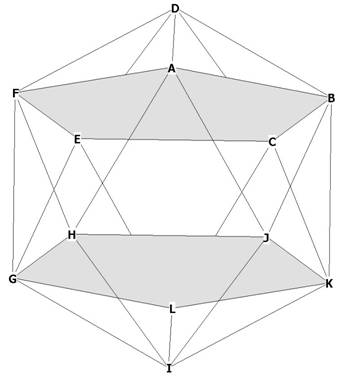Figure 1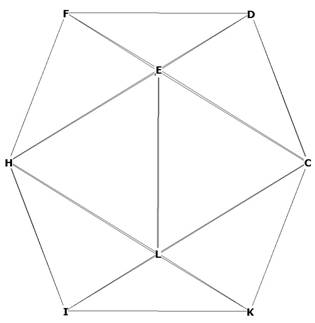Figure 2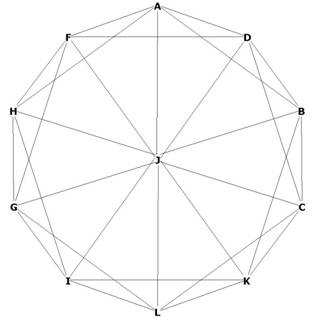Figure 3
Figure 1 shows 2 of the internal pentagons of the icosahedron, LGFDC, and ABKIH.
Figure 2 helps to show that the icosahedron is actually an interlocking series of pentagons. Notice the exterior pentagons at CDFHL, CEHIK, EFHIL, and CDELK.
Figure 3 shows a 2 dimensional 'shadow' of the icosa from the top down. You can see that the outer edges form a perfect decagon, formed by the 2 pentagons CDFGL and ABKIH.
In fact, every vertex of the icosahedron is the vertex of a pentagon.

OK, lets go through the usual analysis and then get on to the interesting stuff!

First. lets calculate the volume of the icosa.
Using the pyramid method we have 20 equilateral triangular faces which serve as the base to a pyramid whose topmost point is the centroid of the icosa.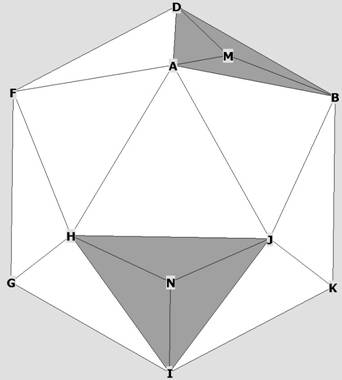Figure 4
Some icosahedron faces with midpoints. All 20 faces will be connected to O to form 20 pyramids.Figure 5    1 icosahedral pyramid

The volume  of each pyramid is 1/3 * area of base * pyramid height.
The area of the base is the area of the equilateral triangle ADB.
The height of the pyramid is OM.
From  The Equilateral Triangle  we know the area is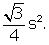All vertices of the icosahedron (as with all 5 of the regular solids) lie upon the surface of a sphere that encloses it. The radius of the circumsphere is O to any vertex, in this case,
r = OA = OB = OD = 1.
The height of the pyramid is h = OM.
In order to find h we need to recognize that any of the triangles OMA, OMB, OMD are right.
This is because OM is perpendicular to the plane of triangle ABD by construction.
AB = BD = AD = side of icosahedron = s.

Lets work with triangle OMD:Figure 6
We know, from  The Equilateral Triangle that DM =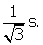In order to find h, we need to find OD = r, in terms of the side s of the icosahedron.
To do that, we have to recognize one of the basic geometric properties of the icosahedron.
Take a look at Figure 1. DI, BG and FK are all diameters of the enclosing sphere around the icosahedron.
Notice rectangle BFGK and notice that BG, FK are both diagonals of it. Now notice that both FB and GK are both diagonals of the 2 pentagonal planes marked in gray.
We know from  Composition of the Pentagon  that the diagonal of a pentagon is* side of pentagon.
Therefore FB = GK =* side of icosahedron, since each side of the pentagon is a side of the icosahedron.

FB =* s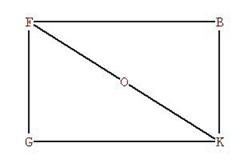Figure 7
FK is the diameter of the enclosing sphere around the icosahedron. OF = OK is the radius r, which we are now trying to find.
FG = BK is the side s of the icosahedron.
We have found that FB = GK =* s .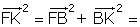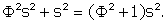FK =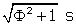, r = d/2 and  r =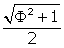s.

Now we can find hthe pyramid height.
Going back to Figure 5 and Figure 6, we can write:
= OM² = r² - DM²  =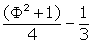=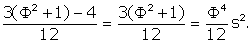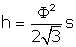is the height of the icosahedron pyramid..
So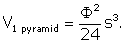There are 20 pyramids, 1 for each face so=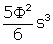=  2.181694991s³.

What is the surface area of the icosahedron?
It is just the area of 1 face * 20 faces. The area of each face is, from above,.
So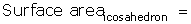We have already noted the relationship between the radius of the enclosing sphere and the side of the icosahedron: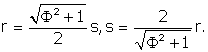r = .951056517s,  s = 1.051462224r.

The side or edge of the icosahedron is slightly larger than the radius.

What is the central angle of the icosahedron?
The central angle,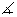DOB, can be seen clearly from Figure 5, and we diagram it in Figure 8 below.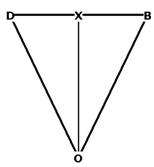Figure 8
OD = OB = r=s. DB is the side of the icosahedron, s.

sin(XOB)  =XOB  =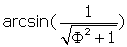=  31.7174744°.DOB = 2 *XOB.
We recognize triangle OXB as our old friend the Phi Right Triangle. From this we know that OX / XB =.
Central angle = 63.4349488°
Surface angles = 60°

What is the dihedral angle of the icosahedron?
The dihedral angle is the angle formed by the intersection of 2 planes:Figure 9
The intersection of the 2 faces DFA and ABD forms the dihedral angle FXB. (See Figure 4 as well).
FX = XB we know, from    to be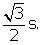FB = a diagonal of one of the pentagons. This can be seen in Figure 1 or Figure 4 as the diagonal of pentagon ABCEF.
Therefore FB =* s and PB =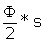.
Triangles XPB and XPF are right by construction, soPXB = 1/2FXB.
sin(PXB) = PB / XB =PXB =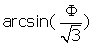= 69.09484258°
Dihedral angle = 138.1896852°

Now lets figure out the distances from the centroid of the icosahedron to any vertex, to any  mid-face, and to any mid-side.
We already have the first two (see Figure 10). OD = OA = OB = r =OM = h =Now we need to find, for example,  OX.Figure 10
If we lay a 3D model of the icosahedron on one of its sides, we can see that a line through the centroid O is perpendicular to that side. So the triangle OXB, for example, is right.
We know AB = s, so XB = (1/2)s. OB = r, so
OX² = OB² - BX²   =OX =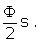distance from centroid to  mid-face == 0.755761314s.
distance from centroid to mid-side ==  0.809016995s.
distance from centroid to a vertex == 0.951056517s.

Comparing distances,  we have  .755761314s,   .809016995s,   .951056517s.

Now lets get to the interesting stuff!
Going back to figure 1, we can see that the icosahedron is composed of interlocking pentagonal 'caps.'
Look at D-ABCEF and I-GHJKL to see this more clearly. Of course , EVERY vertex of the icosahedron is the top of a pentagonal cap, not just D and I.
Let’s analyze this cap: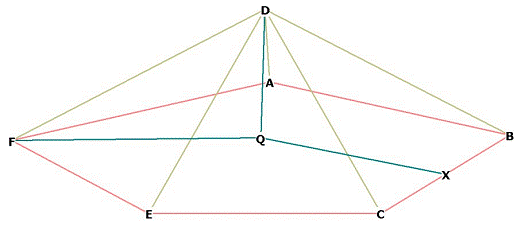Figure  12
Triangle DQF is right, by construction. D is directly over the point Q in the icosahedron.
How far off the plane of pentagon AFECB is D?
In other words, what is the distance DQ?
First, realize there is a circle around pentagon AFECB, even though I haven’t draw it here. QF is the radius of that circle. We know from   Construction of the Pentagon, Part 2 that this radius
r = FQ =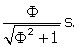Here, s = the side of the icosahedron.
DF is a side of the icosahedron, so DF = s. Therefore we write
DQ² = DF² - FQ²  =DQ =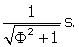Hmmm, this is looking interesting. Lets compare FQ to DQ.
FQ / DQ =In order to form equilateral triangles with D and the other vertices of the pentagon, D has to be raised off the pentagonal plane AFECB by the division of the radius of the pentagon (FQ) in Mean and Extreme Ratio.
The triangle DQF is therefore a Phi based triangle, specifically, a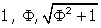triangle. From  The Phi Triangle  we know thatDFQ = 31.71747441°.
Is this surprising? It was to me! I didn't expect something that was composed entirely of equilateral triangles to have any relationship to. An equilateral triangle isgeometry,isgeometry.Figure 13
Here we have Figure 1 basically, with the mid-face points of the 2 pentagons marked off as Q and Z.
We have already seen that DQ is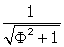with respect to the side of the icosahedron.
That means  IZ =s as well, since IZ = DQ.
What about OQ = OZ? and QZ? How do all of these distances relate to the diameter of the enclosing sphere, DI?

Remember that the distance DI = FK = BG, etc.,  is the diagonal of any of therectangles of which the icosa is composed. One of theserectangles is clearly visible in Figure 12 as BFGK. We know this is arectangle because it is the diagonal of the pentagon ABCEF.
In fact, if you place 3 of these rectangles perpendicular to each other, the 12 corners of the 3 rectangles are the vertices of the icosahedron!

FB =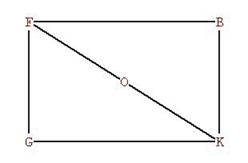Figure 7, repeated
I have copied Figure 7 from above. FK = diameter, OF = radius of enclosing sphere.
We know from above that  d = FK = DI =.
We have already figured out DQ = ZI. So QZ = DI - 2*DQ.
QZ =Now,
QZ / DQ  =So DZ is divided in EMR at Q (See Figure 13).

One interesting fact appears here: FQ = QZ. This means that the distance from one pentagonal plane to the other is precisely the radius of the circle that encloses the pentagon!

What is OQ?
It looks like OQ is just one-half that of QZ, or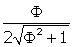s. But is it? Let’s find out.
We know OD = r =from above.
OQ =  OD - DQ  =OQ =s.  We could have gotten this more easily from the fact that

DQ =Yes, OQ is exactly one-half QZ.

Also, OQ / DQ  == 0.951056517

Table of Relationships

Figure 14.  Central axis (diameter) relationships   diameter = DI
(Available in the book).

DZ is divided in EMR by Q,  IQ is divided in EMR by Z.

All of these relationships come from the pentagon!

On the outside of the icosahedron, we see equilateral triangles. But the guts of this polyhedron comes from pentagonal relationships. The equilateral triangles come about from the lifting of the pentagonal 'cap' vertex off the pentagonal plane.
There is now no question that the basis for the construction of the icosahedron is the pentagon.

Or is there?

Take a look at this view of the icosahedron:Figure 15
As you can see, the 2 pentagonal planes in the middle have magically disappeared and become equilateral triangles! All we have done is placed the icosahedron on one of its faces, dropping it from 30° along the x axis and 30° back along the negative y axis.
It seems that all of our work is wrong, except:

The sides of the equilateral triangles EHB and JGC  are all diagonals of pentagons!
EH is a diagonal of pentagon FEJIH, EB is a diagonal of pentagon DEJKB, and HB is a diagonal of pentagon AHIKB.

The equilateral triangle faces of the icosahedron are a by-product of how the pentagons interlock. This is shown in  Figure 16 below: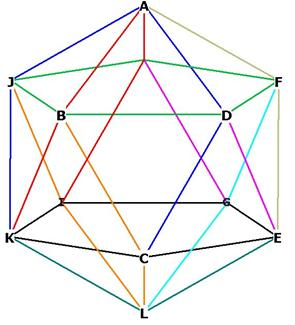Figure 16 --  attempting to show the individual pentagons of the icosahedron

Because the pentagons are interlocking, there is duplication of colors, which makes the individual pentagons a bit difficult to identify.
Begin with the two pentagons BDFHJ, in green, and CEGIK, in black.
Insert pentagon AJKCD, 4 of which sides are in blue.
Insert pentagon ABKIH, 4 of which sides are marked in red.
Insert pentagon BCLIJ, 4 of which sides are marked in orange.
Insert pentagon ADEGH, 2 of which sides are marked in magenta.
Insert pentagon AFECB, 2 of which sides are marked in  gold.
Insert pentagon CDFGL, 2 of which sides are marked in cyan (sky blue)
The side KL in white is part of the pentagon  KLGHJ.
The side LE is part of the pentagon ELIHF.

Icosaahedron Reference Tables
(included in the book)